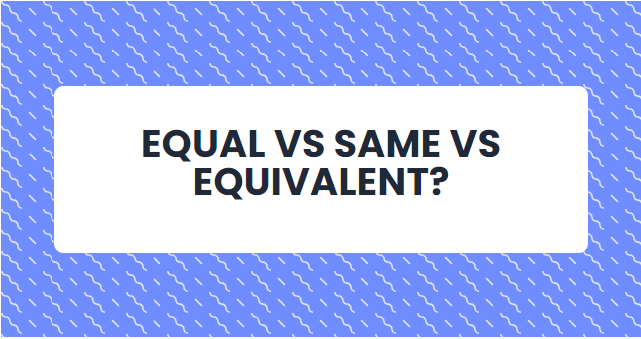# Asserting Equal vs Same vs EquivalentIn this article, I'm going to demonstrate three very common but possibly confusing assertion differences in the world of C# testing:

Let's deep dive into their key differences, with code examples in C# so we get a better understanding of how they behave and differate.

## Assert.AreEqual

Assert.AreEqual checks if two objects have the same value. For value types, this means that their actual values are compared. For reference types, their content determines equality.

`[Test]public void AreEqualExample(){    int value1 = 5;    int value2 = 5;    Assert.AreEqual(value1, value2);}`

This example passes because both have the value 5.

## Assert.AreSame

Assert.AreSame checks if two reference type objects point to the same instance in memory. This does not check if the content of the two objects is the same.

`[Test]public void AreSameExample(){    string str1 = "hello";    string str2 = str1;    Assert.AreSame(str1, str2);}`

This example passes because both point to the same memory instance.

## Should().BeEquivalentTo

FluentAssertions offers another variant of these type of assertions, called Should().BeEquivalentTo() which is especially useful when working with complex objects, like classes.

This method inspects values of two instances and the expectation for equivalency, even if they are of different types.

```public class Person
{
public string Name { get; set; }
public int Age { get; set; }
}

[Test]
public void ShouldBeEquivalentToExample()
{
var person1 = new Person { Name = "John", Age = 25 };
var person2 = new Person { Name = "John", Age = 25 };

person1.Should().BeEquivalentTo(person2);
}```

This example passes because both objects have the same properties and values.

As mentioned, unlike Assert.AreEqual, Should().BeEquivalentTo() does not care if the objects compared are of different types. For instance, two instances created from two completely unrelated classes could be equivalent to one another if they have the same properties and values assigned.

```public class Person
{
public string Name { get; set; }
public int Age { get; set; }
}

public class Student
{    public int Age { get; set; }
public string Name { get; set; }
}

[Test]
public void ShouldBeEquivalentToDifferentTypeExample()
{
var person = new Person { Name = "John", Age = 25 };
var student = new Student { Name = "John", Age = 25 };

person.Should().BeEquivalentTo(student);
}```

This example passes even though they are of different type because both objects have the same properties and values assigned.

Note: As you might have noticed, the order in which properties appear or collections are listed does not matter. They are considered equivalent as long as the content is the same. This is not the case when using other content comparing tools, such as Snapshots, where to order of the properties actually do matter.

## Conclusion

• AreEqual checks for equal values on same reference types.
• AreSame checks for the same instance in memory.
• Should().BeEquivalentTo checks for equivalent content (i.e property values), even across different reference types.

Hope this can be valuable information for you!
Cheers friends! ❤️